# Processing a batch of HL7 messages

When attempting to handle a batch of HL7 messages, customers often ask us why Iguana complains about a missing MSH segment. Following the HL7 Standard, any message must start with an MSH segment; unfortunately, batches of HL7 messages never do. The solution to this problem is easy: simply strip off the batch envelope (FHS BHS … BTS FTS) and process each of contained HL7 messages individually.

Note: You should now use the batch.lua module instead of the code on this page. The code on this pages works exactly the same way, the module simply follows best practice by separating out the two functions `splitBatch()` and `convertTerminators()`. For details see the updated code sample Process a batch of HL7 messages and the module batch.vmd in our code repository.

## Before We Start

Before tackling this problem, you need to understand the difference between a single HL7 message and a batch of messages.

A single HL7 messages consists of a group of segments, starting with the MSH segment (outlined in red):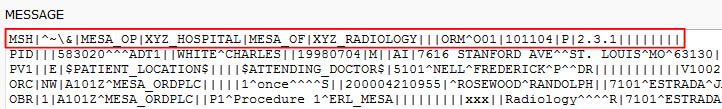A batch messages contains several single HL7 messages (marked by their starting MSH segments). However, the batch itself is identified with FSH and BSH batch headers, not MSH segments.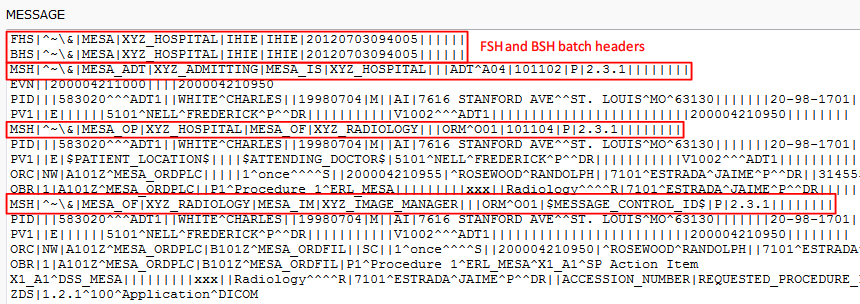Note: These images show the sample data that we use in the example below.

## How It Works

To fully demonstrate the solution, we will first demonstrate the problem. The sample code for each step is included in our explanations so that you can try it out for yourself!

### Demonstrating The Problem

In order to perform some basic mapping tasks, we need to extract data from incoming messages. In this particular case, the incoming message is a batch message.

Our first attempt to process the batch uses the same approach we would use for a single HL7 message:

1. Create a VMD that matches the message structure
2. Parse the message using that VMD with the `hl7.parse()` function

As you can see below, the first attempt to parse the batched message fails, raising a “missing MSH segment” error: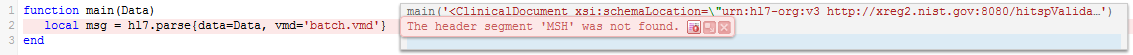So why did this fail? The answer is simple, though perhaps not entirely obvious. It is because `hl7.parse()` follows the HL7 standard, it expects an MSH header segment at the start of the file. As the MSH segment is missing it raises an error.

When we look at the message and the VMD we can see that the first segment will never an MSH segment, hence this approach does not work.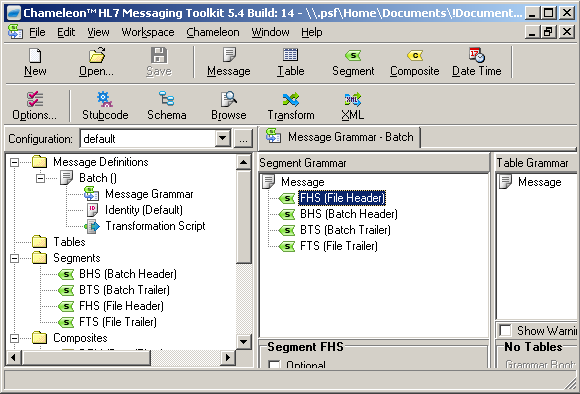#### Sample Code

To reproduce this error, load the code and sample data into a To Translator in the Destination component of a channel.

Use the batch.vmd , the SampleData.txt and the code below:

```function main(Data)
local msg = hl7.parse{data=Data, vmd='batch.vmd'}
end```

### Solving The Problem

Our first attempt to parse the batch as if it was a single message failed, because the first segment is always a batch header (instead of the expected MSH segment).

The solution is to process the messages in the batch “manually”. First we strip off the batch envelope, by removing the FHS, BHS, BTS, and FTS segments. Then we split the data into separate messages, and process each message individually. Below we show you an example of a Lua script that does exactly this.

### How the code works

The code is too long to show in a single screenshot, so we included smaller screenshots for each step. You can see all the code in the Sample Code section below.

The easiest way to use this page is to import the HL7_batch.zip project into a From Translator component (it contains all the code and sample data). Then you can see the code in action while you are reading the explanations.

1. We convert all line terminators to carriage returns “\r”
When we pasted the sample data in Windows it “helpfully” converted the “\r” line breaks to “\n”, hence the need for this step.Note: if you are using Mac OSX or Linux they both use “\r” for line breaks (the same as the HL7 standard), so this issue would not arise.

2. Split the batch into an array of messages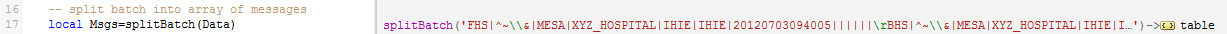The `splitBatch()` function contains several steps
1. Strip the batch headers and footers off the Data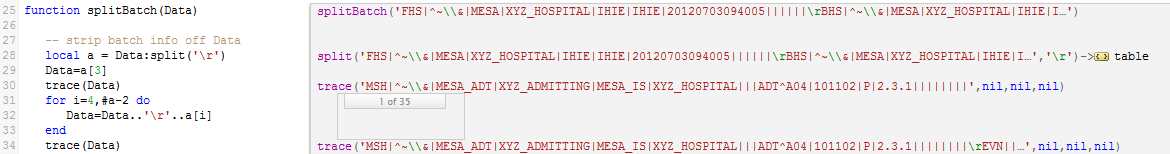As you can see from these annotation dialogs the header and footer segments have been removed: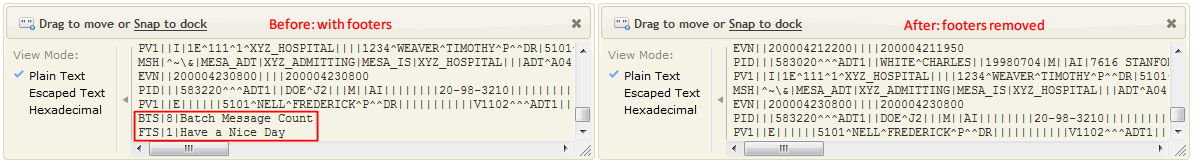2. Split the data into a table of separate messages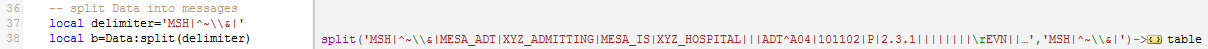This annotation dialog shows the table of separate messages: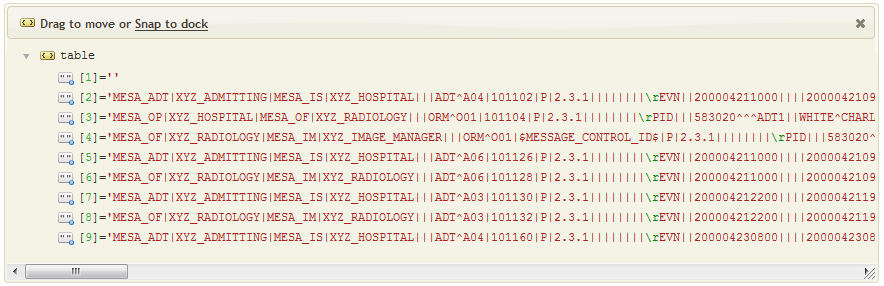The MSH Segment information is missing, we add this to the beginning of each message in the next step. Also we “renumber” the messages correctly from 1 to 8.
3. Add the MSH segment information to each messageThe code in this step is a little bit clever:
• It starts at index 2 of the table so it does process the empty first row
• It copies each message to the previous row, to correct the numbering
• As it copies, it also prepends the MSH Segment information
• Finally it deletes the last row which contains a redundant (partial copy of the) last message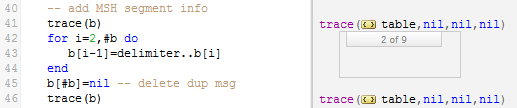This annotation dialog shows that the table now contains 8 messages that include MSH Segment data: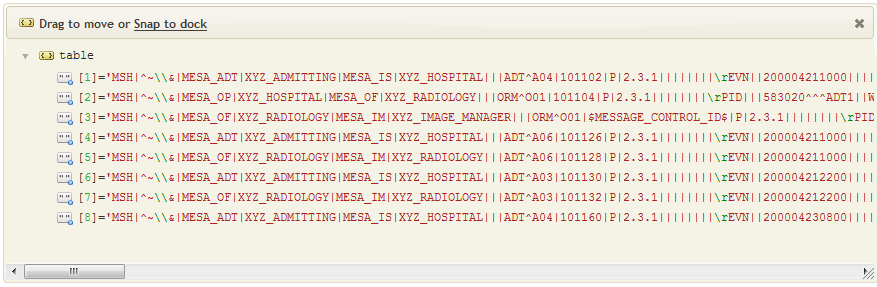4. Some ideas that could potentially be useful when processing a batch
• We could create a global variable MsgCnt to count the number of messages in the batch. This could be used to check the message count stored in the BTS segment. If it does not match an error could be raised and/or processing of the batch aborted.
• We could make global copies of the headers and footers. This might be useful if for logging information identifying which batches were processed, etc.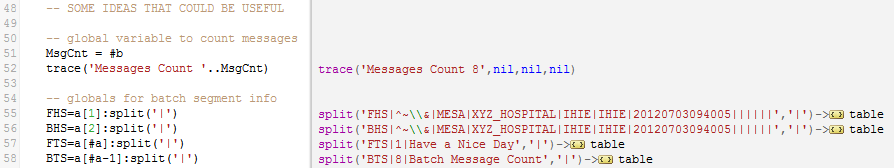5. Finally we return the the table containing the separate messages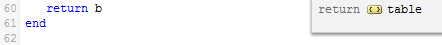3. Process the each message in the table of messages individually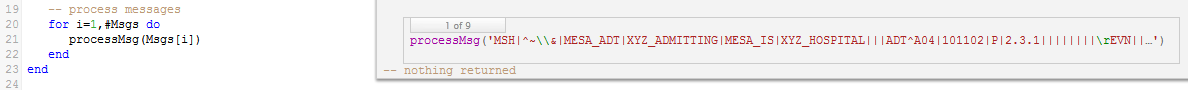The `processMsg()` function call the call the `MapData()` function, and then writes data to the database (comment only):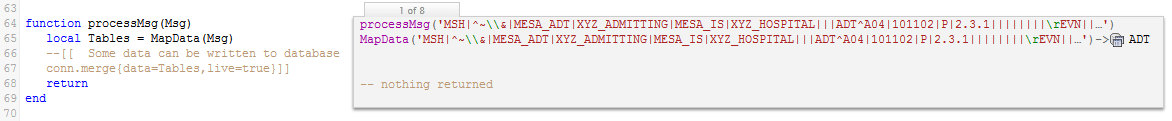The `MapData()` function creates an HL7 message tree the “out” table tree, and then maps data to “out” (comment only):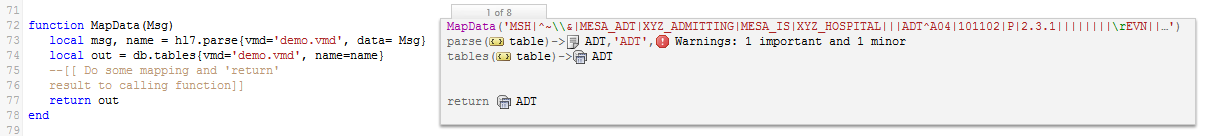#### Sample Code

Import the HL7_batch.zip project into a From Translator component, it contains all the code and sample data.

Alternatively paste the code from below into a From Translator, use the SampleData.txt and also the latest stringutil module from our code repository:

```-- this code follows best practice by using local
-- functions first followed by main() at the end

require'stringutil'

conn = db.connect{
api=db.MY_SQL,
name='test',
user='',
live=true}

local function splitBatch(Data)

-- strip batch info off Data
local a = Data:split('\r')
Data=a
trace(Data)
for i=4,#a-2 do
Data=Data..'\r'..a[i]
end
trace(Data)

-- split Data into messages
local delimiter='MSH|^~\\&|'
local b=Data:split(delimiter)

trace(b)
for i=2,#b do
b[i-1]=delimiter..b[i]
end
b[#b]=nil -- delete dup msg
trace(b)

-- SOME IDEAS THAT COULD BE USEFUL

-- global variable to count messages
MsgCnt = #b
trace('Messages Count '..MsgCnt)

-- globals for batch segment info
FHS=a:split('|')
BHS=a:split('|')
FTS=a[#a]:split('|')
BTS=a[#a-1]:split('|')

return b
end

local function MapData(Msg)
local msg, name = hl7.parse{vmd='example/demo.vmd', data= Msg}
local out = db.tables{vmd='example/demo.vmd', name=name}
--[[ Do some mapping and 'return'
result to calling function]]
return out
end

function processMsg(Msg)
local Tables = MapData(Msg)
--[[  Some data can be written to database
conn.merge{data=Tables,live=true}]]
return
end

local function convertTerminators(Data)
Data = Data:gsub('\r\n','\r')
return Data:gsub('\n','\r')
end

function main(Data)
-- convert non-conformant terminators to "\r"
Data = convertTerminators(Data)

-- split batch into array of messages
local Msgs=splitBatch(Data)

-- process messages
for i=1,#Msgs do
processMsg(Msgs[i])
end
end```